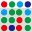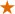#### File Exchange > Data Analysis >    Bootstrap SamplingAuthor:
OriginLab Technical Support
6/24/2022
Last Update:
11/3/2022
278
Total Ratings:
5
File Size:
220 KB
Average Rating:File Name:
Bootstrap_...ng.opx
File Version:
1.00
Minimum Versions:
Summary:

Use bootstrap sampling to estimate the statistic of input data.

Description:

PURPOSE
This app can be used to estimate the standard error and confidence interval of a column’s statistic, correlation coefficient of two columns and coefficients of multiple linear regression by bootstrap sampling.

INSTALLATION
Download the file Bootstrap_Sampling.opx, and then drag-and-drop onto the Origin workspace. An icon will appear in the Apps Gallery window.
NOTE: This tool requires OriginPro.

OPERATION

1. Click Bootstrap Sampling icon in the Apps Gallery window.
2. In the opened dialog, choose a type in Analysis Type radio group. Three types are available: Statistics on ColumnCorrelation Coefficient and Multiple Regression. Select input data.
3. In the Settings tab, set Number of Bootstrap Samples. And choose a Statistic to estimate, options include Descriptive Statistics and Custom. When Custom is chosen, type a LabTalk function name to calculate the statistic in Custom Function edit box. In the Bootstrap Standard Error and Confidence Interval of Statistic group, choose whether to calculate Standard Error and Confidence Interval. When Confidence Interval is chosen, set Confidence Level and select a method. Three methods are available: PercentileBasic (reverse percentile) and BCa (bias-corrected and accelerated). Check Histogram will create a histogram graph for the statistic of bootstrap samples.
4. Click OK button. A report with the Statistics table and Histogram graph will appear.

SAMPLE OPJU FILE
This app provides a sample OPJU file. Right click on the Bootstrap Sampling icon in the Apps Gallery window, and choose Show Samples Folder from the short-cut menu. A folder will open. Drag-and-drop the project file BSEx.opju from the folder onto Origin. The Notes window in the project shows detailed steps.
Note: If you wish to save the OPJU after changing, it is recommended that you save to a different folder location (e.g. User Files Folder).

ALGORITHM
Bootstrapping uses random sampling with replacement for column data. It can estimate standard error and confidence interval for the statistic. For regression data, it uses random sampling with residuals.

• Confidence Interval
• Percentile method
upper limit, $$1-(1-\alpha)/2$$ percentile, lower limit, $$(1-\alpha)/2$$ percentile
• Basic method, also known as reverse percentile
upper limit, $$2Value - (1-\alpha)/2$$ percentile, lower limit, $$2Value - (1-(1-\alpha)/2)$$ percentile, where Value is the statistic.
• BCa (bias-corrected and accelerated) method
• Compute the bootstrap statistic $$\hat{\theta}_b$$.
• Calculate the bias correction $$\hat{Z}_0$$.
• Use jackknife resampling method to calculate the acceleration factor $$\hat{a}$$.
• Correct percents for lower and upper confidence limits with $$\hat{Z}_0$$ and $$\hat{a}$$, for $$100( 1-\alpha )\%$$ confidence interval:
$$\alpha_1 = \Phi( \hat{Z}_0 + \frac{ \hat{Z}_0 + Z_{(\alpha/2)}}{ 1 - \hat{a} ( \hat{Z}_0 + Z_{(\alpha/2)} ) } )$$
$$\alpha_2 = \Phi( \hat{Z}_0 + \frac{ \hat{Z}_0 + Z_{(1-\alpha/2)}}{ 1 - \hat{a} ( \hat{Z}_0 + Z_{(1-\alpha/2)} ) } )$$
where  $$\Phi$$ is the cumulative distribution function of standard normal, and $$Z_{\alpha}$$ is the $$100 \alpha$$-th percentile of standard normal.
• Calculate confidence interval by $$100 \alpha_1$$-th and $$100 \alpha_2$$-th percentiles of $$\hat{\theta}_b$$.

REFERENCE

1. Nathaniel E. Helwig, "Bootstrap Confidence Intervals", http://users.stat.umn.edu/~helwig/notes/bootci-Notes.pdf

 05/07/2023 OriginLab Hi mark.fry, Which values you want to estimate in ANOVA? Thanks, OriginLab Technical Service 05/05/2023 mark.fry Really useful. It would be great if this feature could be extended to include bootstrapping ANOVA and KW-ANOVA12/15/2022 marco.carrer Extremely useful app. It would be even better if computing correlation you could select multiple columns (variables).11/13/2022 OriginLab Hi, liudejun The app does not output sample and we did not use CV. You can get the sample mean in the PlotData sheet if you select Descriptive Statistics as output OriginLab Techinical Service 11/13/2022 liudejun can we set sample size and get its samples, sample CVs, sample means？